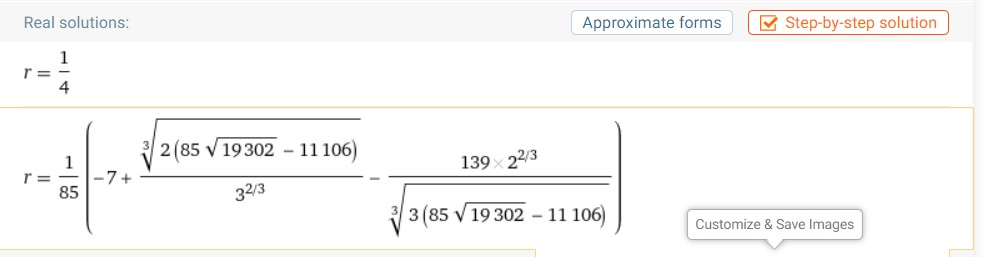# Roots

Solve for $$r:$$ $$\frac{1}{r}$$+$$\frac{1}{r^{2}}$$+$$\frac{1}{r^{3}}$$+$$\frac{1}{r^{4}}$$$$=340$$

I was only able to solve $r=\frac{1}{4}$ but WolframAlpha has $r ≈ -0.221$, which I don't know how to get.Note by Ryan Merino
1 month, 1 week ago

This discussion board is a place to discuss our Daily Challenges and the math and science related to those challenges. Explanations are more than just a solution — they should explain the steps and thinking strategies that you used to obtain the solution. Comments should further the discussion of math and science.

When posting on Brilliant:

• Use the emojis to react to an explanation, whether you're congratulating a job well done , or just really confused .
• Ask specific questions about the challenge or the steps in somebody's explanation. Well-posed questions can add a lot to the discussion, but posting "I don't understand!" doesn't help anyone.
• Try to contribute something new to the discussion, whether it is an extension, generalization or other idea related to the challenge.
• Stay on topic — we're all here to learn more about math and science, not to hear about your favorite get-rich-quick scheme or current world events.

MarkdownAppears as
*italics* or _italics_ italics
**bold** or __bold__ bold
- bulleted- list
• bulleted
• list
1. numbered2. list
1. numbered
2. list
Note: you must add a full line of space before and after lists for them to show up correctly
paragraph 1paragraph 2

paragraph 1

paragraph 2

[example link](https://brilliant.org)example link
> This is a quote
This is a quote
    # I indented these lines
# 4 spaces, and now they show
# up as a code block.

print "hello world"
# I indented these lines
# 4 spaces, and now they show
# up as a code block.

print "hello world"
MathAppears as
Remember to wrap math in $$ ... $$ or $ ... $ to ensure proper formatting.
2 \times 3 $2 \times 3$
2^{34} $2^{34}$
a_{i-1} $a_{i-1}$
\frac{2}{3} $\frac{2}{3}$
\sqrt{2} $\sqrt{2}$
\sum_{i=1}^3 $\sum_{i=1}^3$
\sin \theta $\sin \theta$
\boxed{123} $\boxed{123}$

## Comments

Sort by:

Top Newest
- 1 month, 1 week ago

Log in to reply

Neat! I haven't worked much with cubics, obviously. I didn't understand the end of the second example though:

$y = s-t = \sqrt{10 + 6\sqrt{3}} - \sqrt{-10 + 6\sqrt{3}} = 2$

Is there something I'm missing?

- 1 month, 1 week ago

Log in to reply

$\dfrac8{t^3} - t^3 = 20$

$(\tfrac2t - t)^3 + 3(\tfrac2t - t)\cdot \tfrac2t \cdot t = 20$

$(\tfrac2t - t)^3 + 6(\tfrac2t - t) = 20$

Let $z = \tfrac2t - t$, then $z^3 + 6z = 20$

Rational root theorem tells us that $z=2$ is a root and the other two roots are not real.

so $z = \tfrac2t - t = 2$ only.

Or $s - t = 2$ only.

- 1 month, 1 week ago

Log in to reply

Interesting...the wiki didn't include any of those steps. Would you mind detailing how you went from the first equation to the second? Thanks!

- 1 month, 1 week ago

Log in to reply

Use $a^3 - b^3 = (a - b)^3 + 3ab(a - b)$

- 1 month, 1 week ago

Log in to reply

Cool. Thanks!

- 1 month, 1 week ago

Log in to reply

Thanks. Didn't know this method 'til now.

- 1 month ago

Log in to reply

Hmm... seems complicated. :)- 1 month, 1 week ago

Log in to reply

×

Problem Loading...

Note Loading...

Set Loading...